## Classification

#### Classification

Direction: In each problem, out of the five figures marked (a), (b), (c), (d) and (e), four are similar in a certain manner. However one figure is not like the other four. Choose the figure which is different from the rest.

1. Choose the figure which is different from the rest.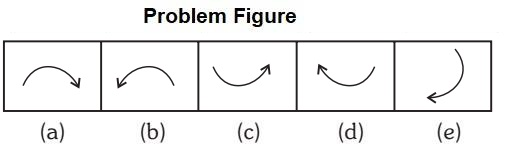1. All are horizontal element except one.

##### Correct Option: E

Rest all are horizontal element except (e) which is vertical

1. Choose the figure which is different from the rest.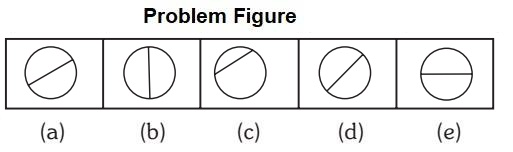1. Every figure has diameter except one.

##### Correct Option: C

Only c is not having diameter.

1. Choose the figure which is different from the rest.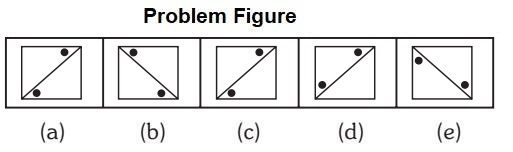1. Dots are on other side of the line.

##### Correct Option: D

All except (d) dots are on other side of the line.

1. Choose the figure which is different from the rest.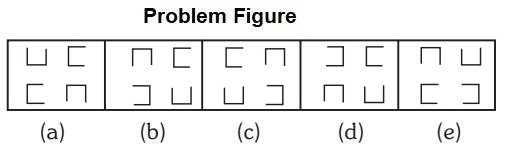1. Two of the four elements are oriented in the same direction.

##### Correct Option: A

Only in figure (a) two of the four elements are oriented in the same direction.

1. Choose the figure which is different from the rest.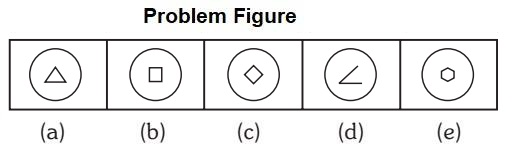1. Inner figures are enclosed.

##### Correct Option: D

All inner figures are enclosed one except (d)